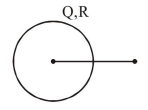# Consider the force

Question:

Consider the force $\mathrm{F}$ on a charge ' $\mathrm{q}$ ' due to a uniformly charged spherical shell of radius $R$ carrying charge Q distributed uniformly over it. Which one of the following statements is true for $\mathrm{F}$, if ' $\mathrm{q}$ ' is placed at distance $\mathrm{r}$ from the centre of the shell ?

1. $\mathrm{F}=\frac{1}{4 \pi \varepsilon_{0}} \frac{\mathrm{Qq}}{\mathrm{r}^{2}}$ for $\mathrm{r}>\mathrm{R}$

2. $\frac{1}{4 \pi \varepsilon_{0}} \frac{\mathrm{q} \mathrm{Q}}{\mathrm{R}^{2}}>\mathrm{F}>0$ for $\mathrm{r}<\mathrm{R}$

3. $\mathrm{F}=\frac{1}{4 \pi \varepsilon_{0}} \frac{\mathrm{Qq}}{\mathrm{r}^{2}}$ for all $\mathrm{r}$

4. $\mathrm{F}=\frac{1}{4 \pi \varepsilon_{0}} \frac{\mathrm{Qq}}{\mathrm{R}^{2}}$ for $\mathrm{r}<\mathrm{R}$

Correct Option: 1

Solution:

Inside the shell

$\mathrm{E}=0$

hence $F=0$

Oustside the sheell$E=\frac{1}{4 \pi \varepsilon_{0}} \frac{Q}{r^{2}}$

hence $\mathrm{F}=\frac{1}{4 \pi \varepsilon_{0}} \frac{\mathrm{Qq}}{\mathrm{r}^{2}}$ for $\mathrm{r}>\mathrm{R}$